Скачать презентацию The Arbitrage Theorem Henrik Jönsson Mälardalen University Sweden

1cc5899f9ebc642949ac32b6dfa81146.ppt

• Количество слайдов: 25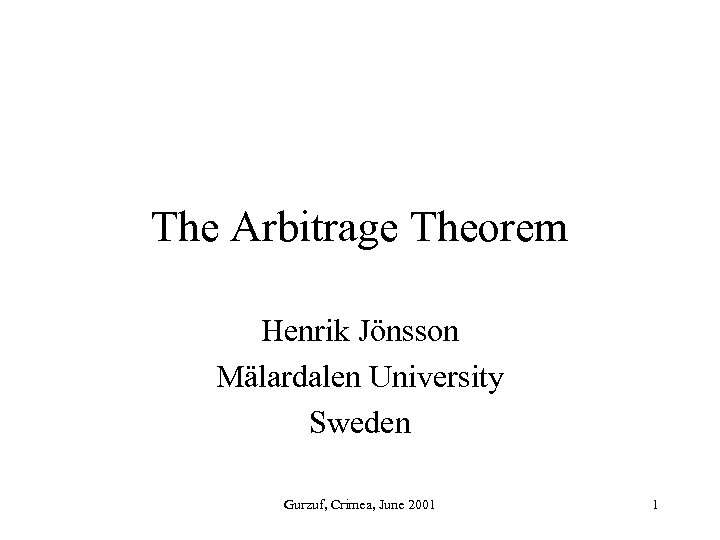The Arbitrage Theorem Henrik Jönsson Mälardalen University Sweden Gurzuf, Crimea, June 2001 1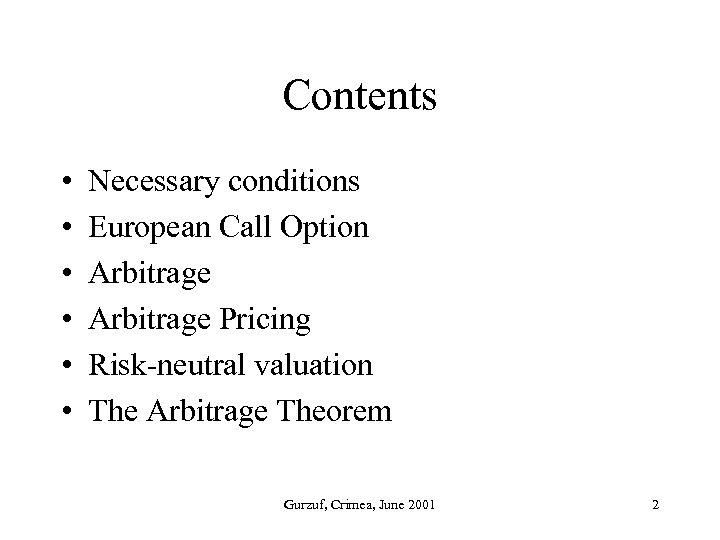Contents • • • Necessary conditions European Call Option Arbitrage Pricing Risk-neutral valuation The Arbitrage Theorem Gurzuf, Crimea, June 2001 2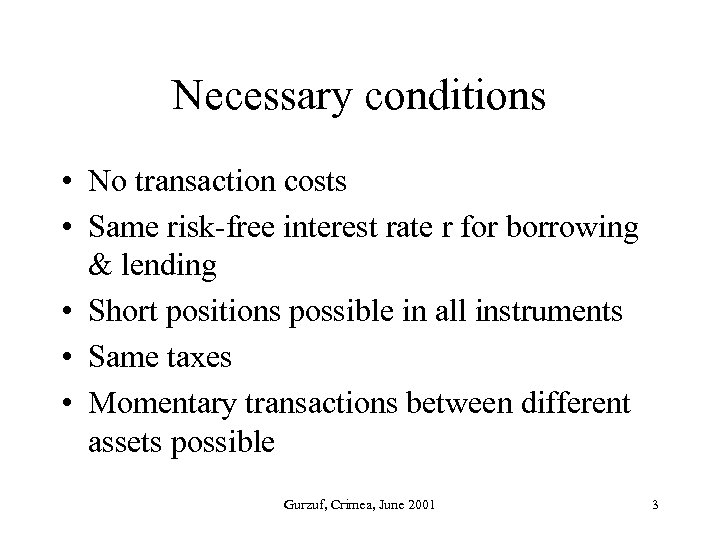Necessary conditions • No transaction costs • Same risk-free interest rate r for borrowing & lending • Short positions possible in all instruments • Same taxes • Momentary transactions between different assets possible Gurzuf, Crimea, June 2001 3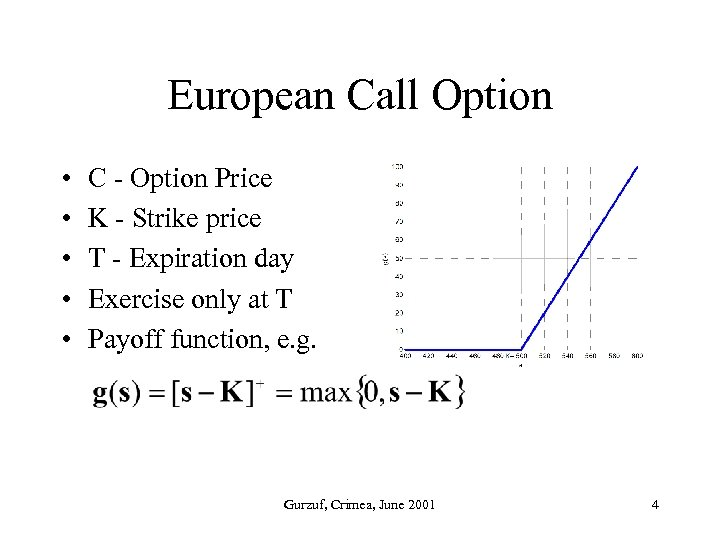European Call Option • • • C - Option Price K - Strike price T - Expiration day Exercise only at T Payoff function, e. g. Gurzuf, Crimea, June 2001 4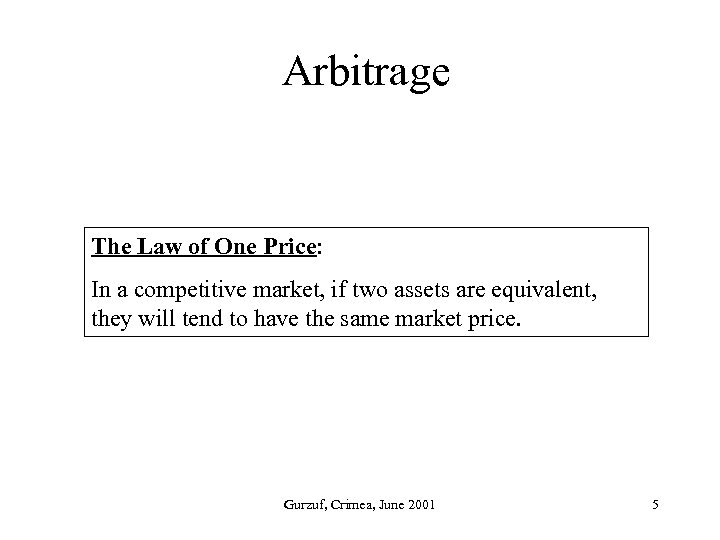Arbitrage The Law of One Price: In a competitive market, if two assets are equivalent, they will tend to have the same market price. Gurzuf, Crimea, June 2001 5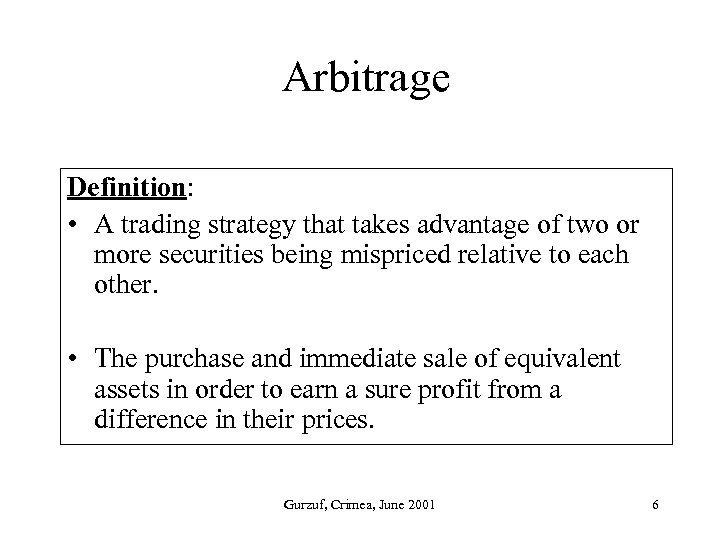Arbitrage Definition: • A trading strategy that takes advantage of two or more securities being mispriced relative to each other. • The purchase and immediate sale of equivalent assets in order to earn a sure profit from a difference in their prices. Gurzuf, Crimea, June 2001 6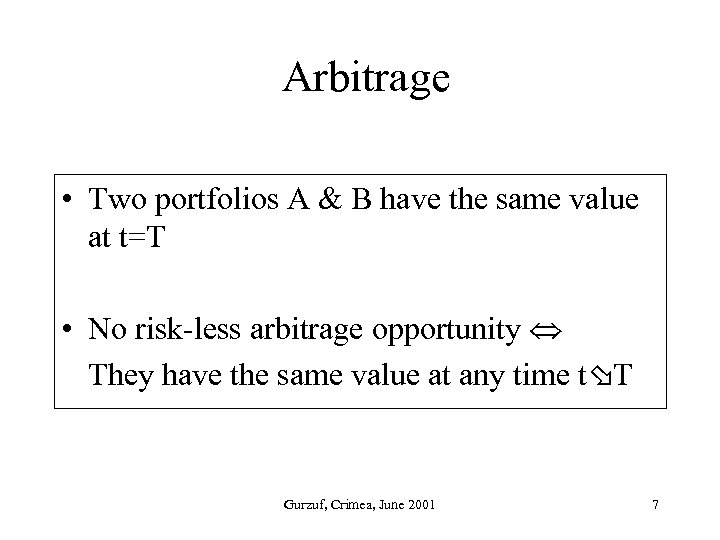Arbitrage • Two portfolios A & B have the same value at t=T • No risk-less arbitrage opportunity They have the same value at any time t T Gurzuf, Crimea, June 2001 7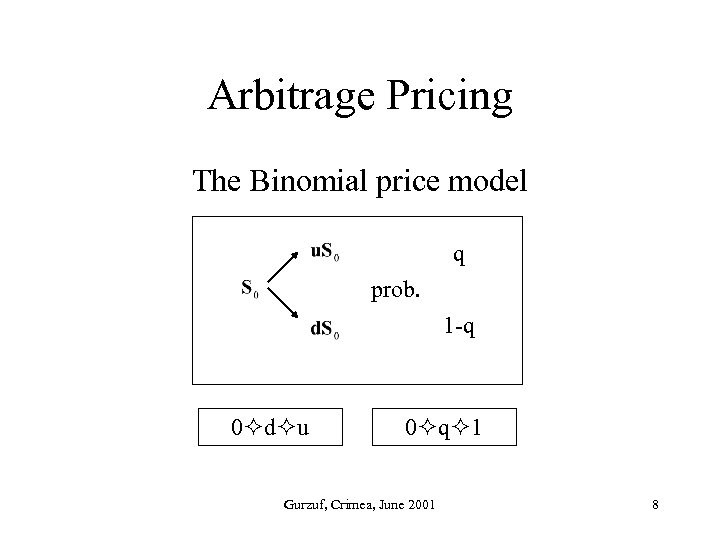Arbitrage Pricing The Binomial price model q prob. 1 -q 0 d u 0 q 1 Gurzuf, Crimea, June 2001 8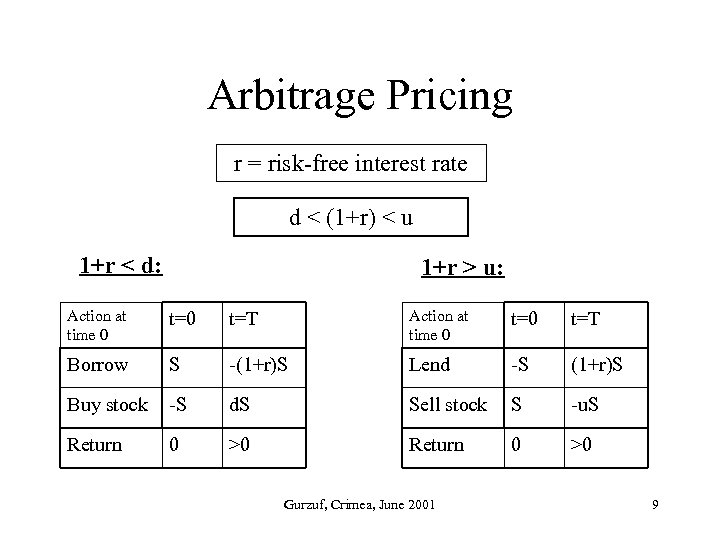Arbitrage Pricing r = risk-free interest rate d < (1+r) < u 1+r < d: 1+r > u: Action at time 0 t=0 t=T Borrow S -(1+r)S Lend -S (1+r)S Buy stock -S d. S Sell stock S -u. S Return 0 >0 Gurzuf, Crimea, June 2001 9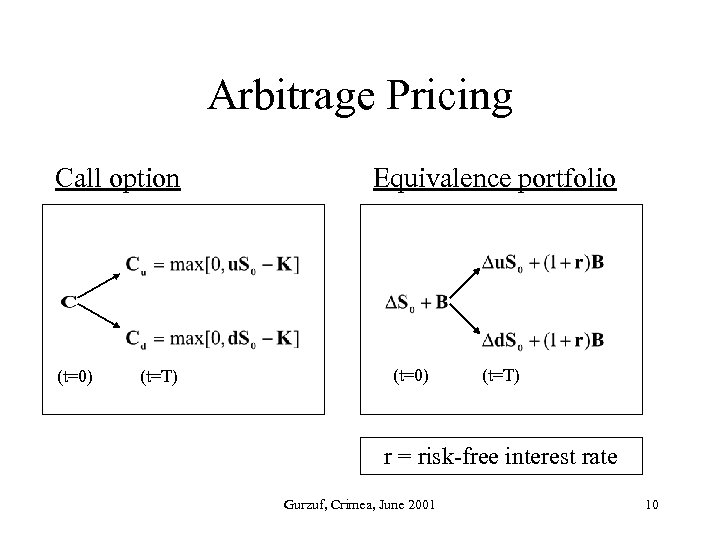Arbitrage Pricing Call option (t=0) (t=T) Equivalence portfolio (t=0) (t=T) r = risk-free interest rate Gurzuf, Crimea, June 2001 10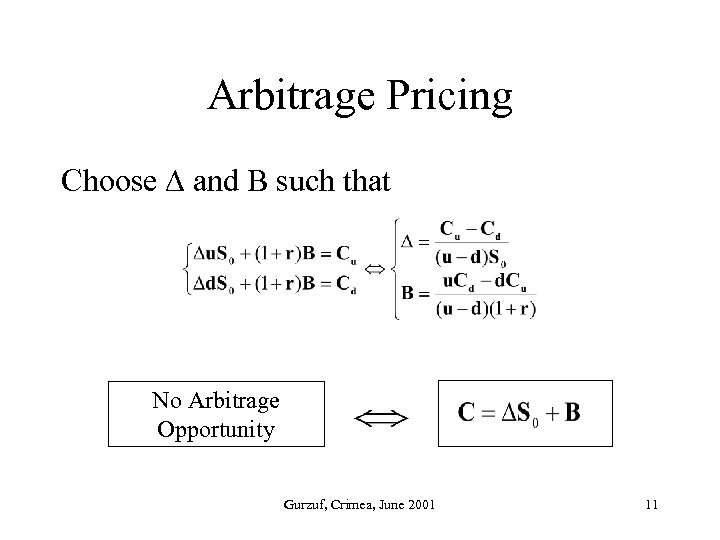Arbitrage Pricing Choose and B such that No Arbitrage Opportunity Gurzuf, Crimea, June 2001 11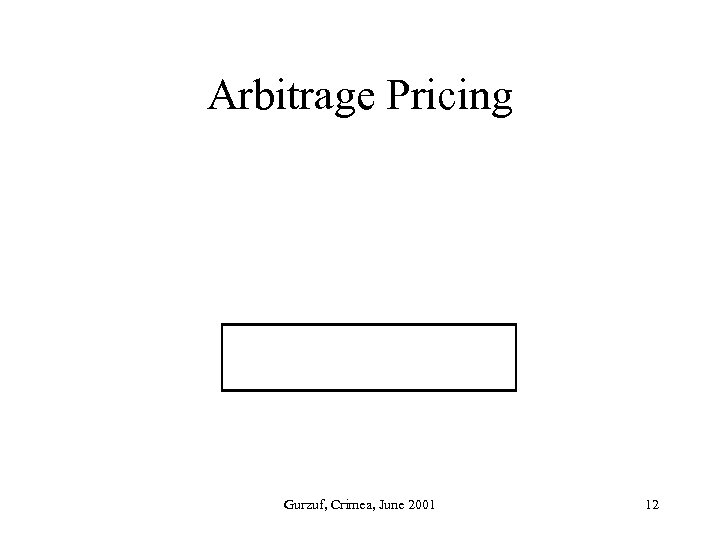Arbitrage Pricing Gurzuf, Crimea, June 2001 12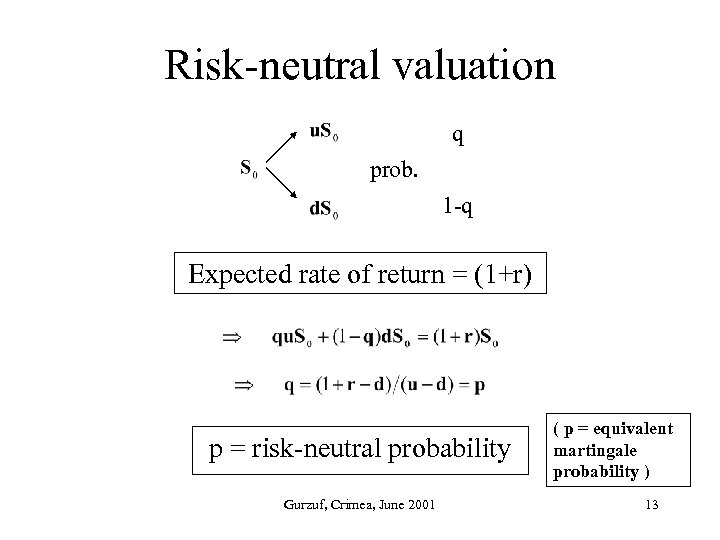Risk-neutral valuation q prob. 1 -q Expected rate of return = (1+r) p = risk-neutral probability Gurzuf, Crimea, June 2001 ( p = equivalent martingale probability ) 13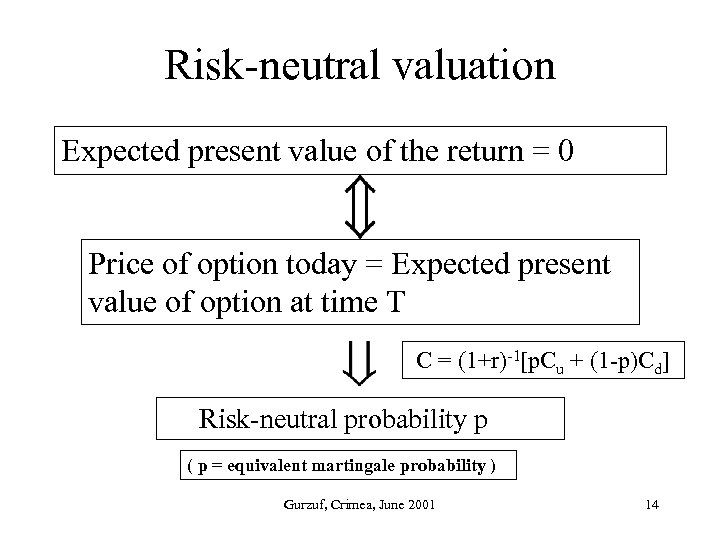Risk-neutral valuation Expected present value of the return = 0 Price of option today = Expected present value of option at time T C = (1+r)-1[p. Cu + (1 -p)Cd] Risk-neutral probability p ( p = equivalent martingale probability ) Gurzuf, Crimea, June 2001 14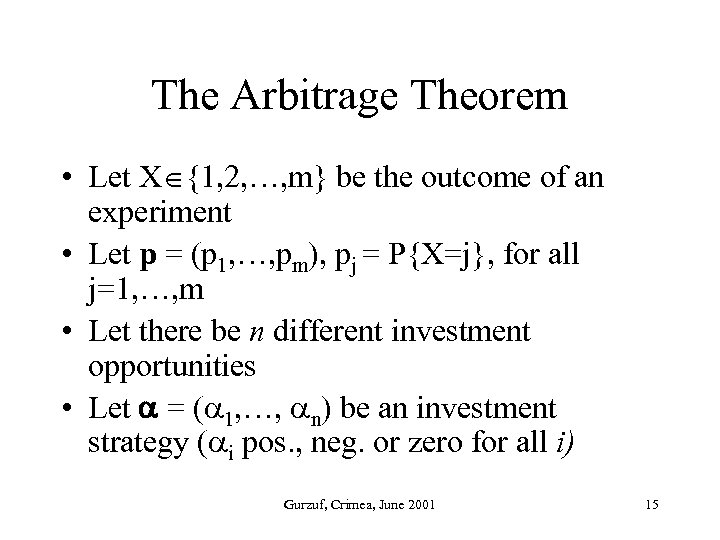The Arbitrage Theorem • Let X {1, 2, …, m} be the outcome of an experiment • Let p = (p 1, …, pm), pj = P{X=j}, for all j=1, …, m • Let there be n different investment opportunities • Let = ( 1, …, n) be an investment strategy ( i pos. , neg. or zero for all i) Gurzuf, Crimea, June 2001 15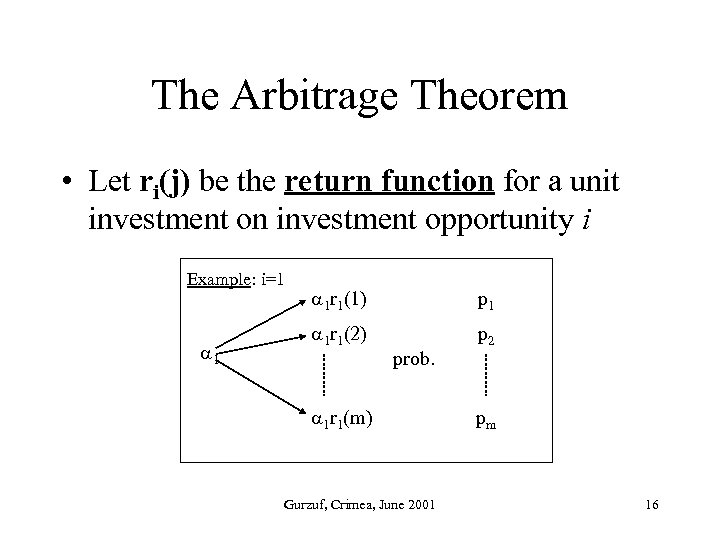The Arbitrage Theorem • Let ri(j) be the return function for a unit investment on investment opportunity i Example: i=1 1 1 r 1(1) p 1 1 r 1(2) p 2 prob. 1 r 1(m) Gurzuf, Crimea, June 2001 pm 16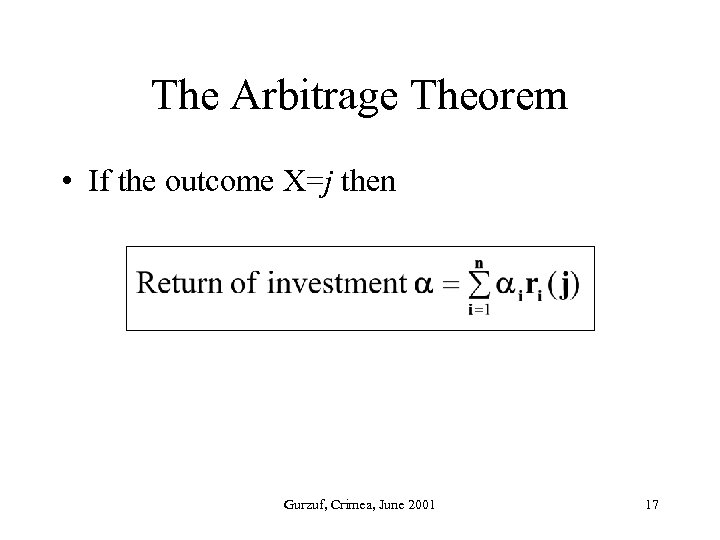The Arbitrage Theorem • If the outcome X=j then Gurzuf, Crimea, June 2001 17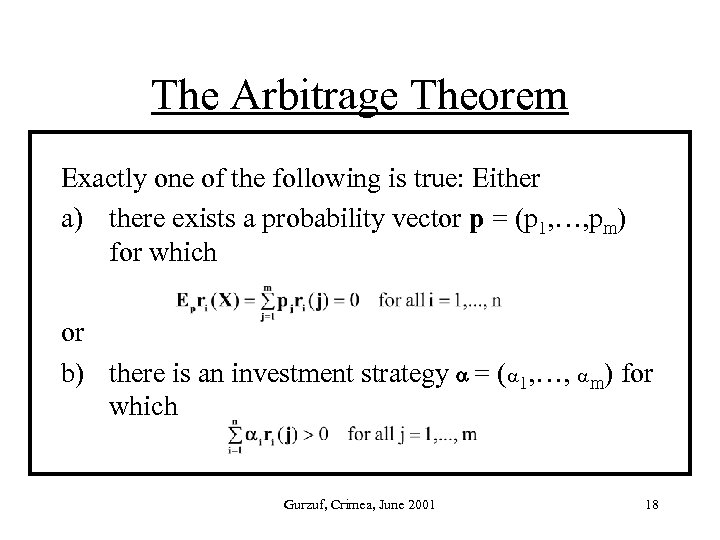The Arbitrage Theorem Exactly one of the following is true: Either a) there exists a probability vector p = (p 1, …, pm) for which or b) there is an investment strategy = ( 1, …, m) for which Gurzuf, Crimea, June 2001 18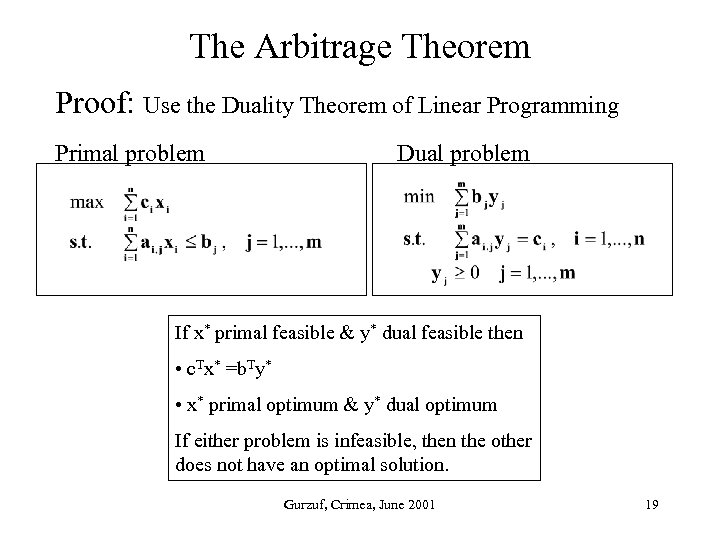The Arbitrage Theorem Proof: Use the Duality Theorem of Linear Programming Primal problem Dual problem If x* primal feasible & y* dual feasible then • c. Tx* =b. Ty* • x* primal optimum & y* dual optimum If either problem is infeasible, then the other does not have an optimal solution. Gurzuf, Crimea, June 2001 19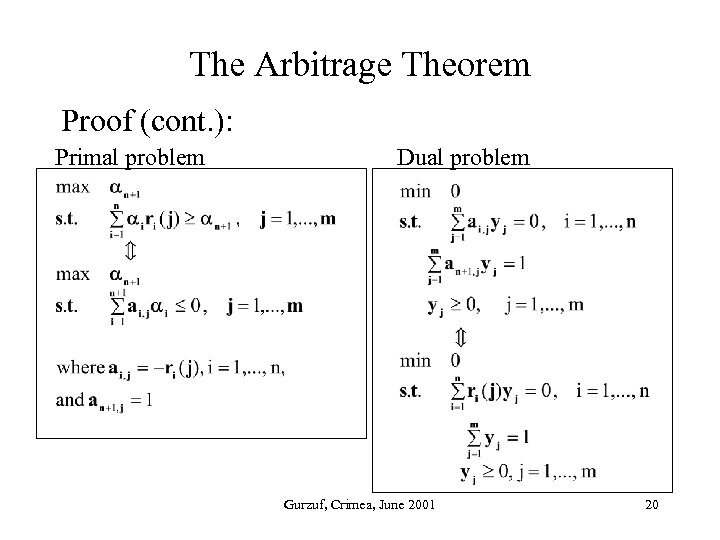The Arbitrage Theorem Proof (cont. ): Primal problem Dual problem Gurzuf, Crimea, June 2001 20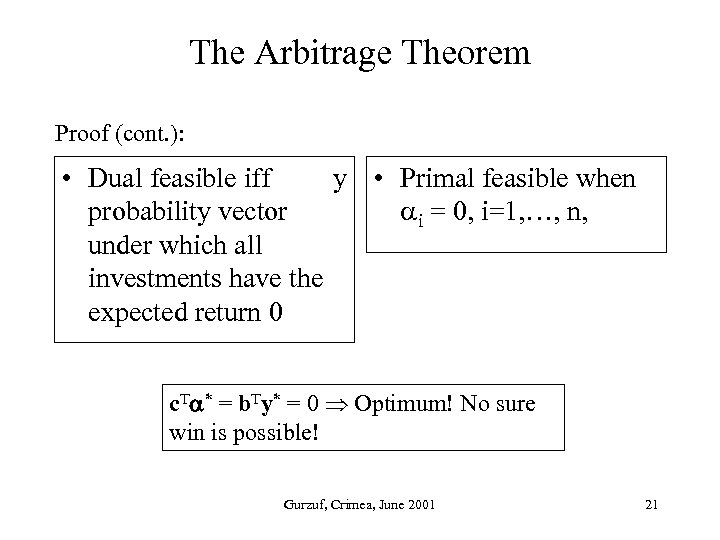The Arbitrage Theorem Proof (cont. ): • Dual feasible iff y • Primal feasible when probability vector i = 0, i=1, …, n, under which all investments have the expected return 0 c. T * = b. Ty* = 0 Optimum! No sure win is possible! Gurzuf, Crimea, June 2001 21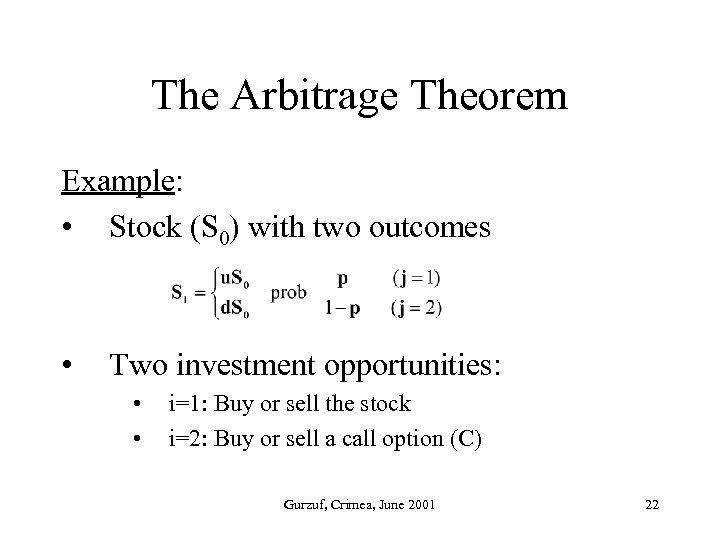The Arbitrage Theorem Example: • Stock (S 0) with two outcomes • Two investment opportunities: • • i=1: Buy or sell the stock i=2: Buy or sell a call option (C) Gurzuf, Crimea, June 2001 22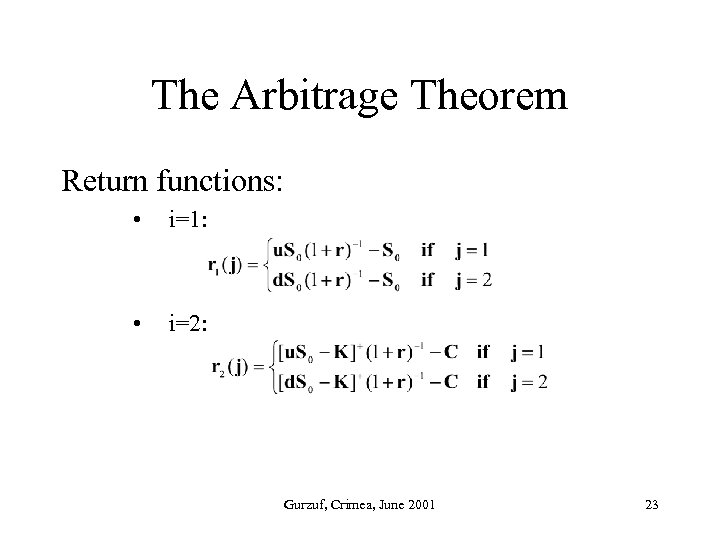The Arbitrage Theorem Return functions: • i=1: • i=2: Gurzuf, Crimea, June 2001 23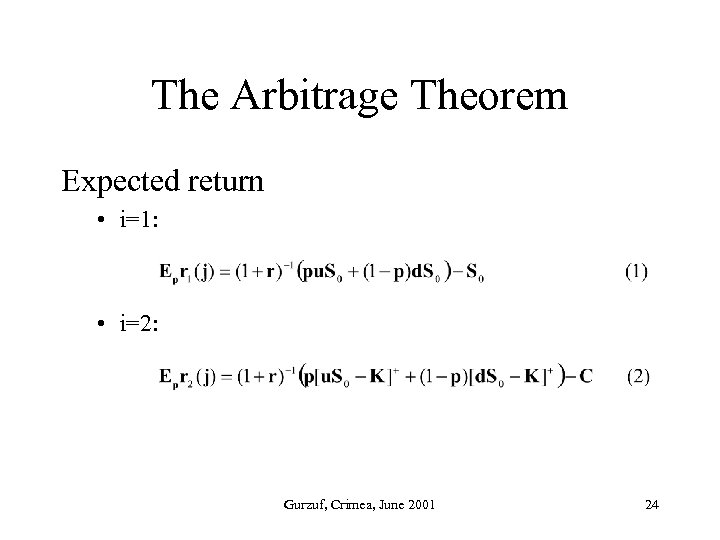The Arbitrage Theorem Expected return • i=1: • i=2: Gurzuf, Crimea, June 2001 24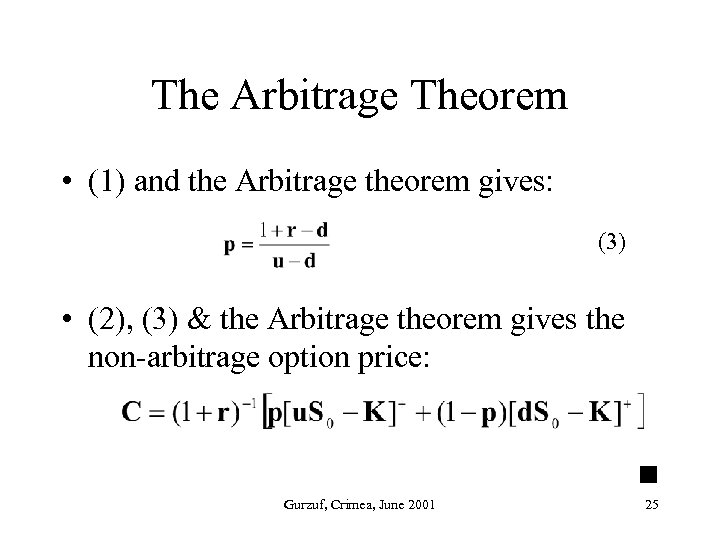The Arbitrage Theorem • (1) and the Arbitrage theorem gives: (3) • (2), (3) & the Arbitrage theorem gives the non-arbitrage option price: Gurzuf, Crimea, June 2001 25